# NMath User's Guide

35.1 Encapsulating Differential Equations (.NET, C#, CSharp, VB, Visual Basic, F#)

Class represents a first order initial value differential equation. If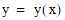is the unknown function, the first order initial value problem may be stated as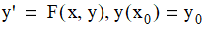wheredenotes the first derivative of y with respect to x, F is a continuous function with bounded partial derivatives, and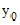is the value of the unknown function y at the point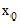.

A FirstOrderInitialValueProblem instance is constructed from a function, F, and initial value,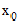and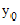. The function F is encapsulated as a Func<double, double, double>, a delegate which takes two doubles and returns a double.

For example, the following code constructs a FirstOrderInitialValueProblem where:

Code Example – C# ordinary differential equations (ODE)

```Func<double, double, double> f =
```
```  delegate( double x, double y )
```
```  {
```
```    return x * x;
```
```  };

```

```double x0 = 0.0;
```
```double y0 = 1.0;

```

```FirstOrderInitialValueProblem prob =
```
```  new FirstOrderInitialValueProblem( f, x0, y0 );
```

Top

Top